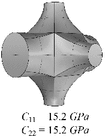# Table 2 Exemplary look-up table for the effective elasticity tensors (here represented by $$C_{11}$$ and $$C_{22}$$) for changing diameters of the rod in x-direction and rotations around the z-axis
Rotation around the $$\mathbf{z}$$ -axis
$$\mathbf{0} ^{\circ }$$ $$\mathbf{22}.5 ^{\circ }$$ $$\mathbf{45} ^{\circ }$$
0.2 mm0.3 mm0.4 mm